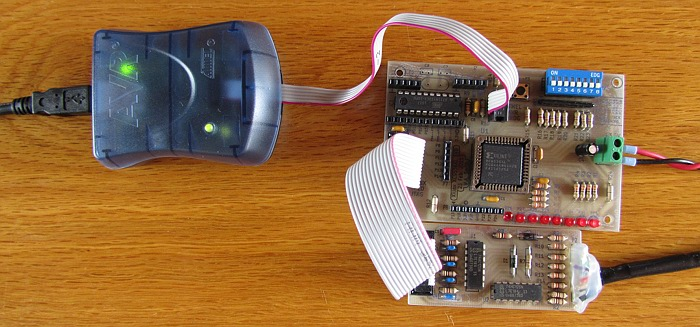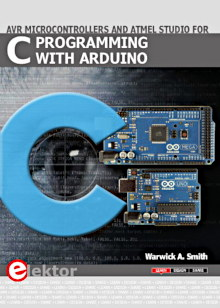Starting Electronics needs your help! Please make a donation to help cover our hosting and other costs. Click the donate button to send a donation of any amount.# Tutorial 6: Clock Divider in VHDL

Created on: 8 January 2012

In this tutorial a clock divider is written in VHDL code and implemented in a CPLD. One LED on the CPLD board is connected to the clock source which is running at about 130Hz, making the LED appear to be switched on. A second LED is connected to the clock source divided by 32 which results in the LED flashing on and off at about 4Hz.

This video shows the VHDL clock divider implemented on a CPLD.

Can't see the video? View on YouTube →

Up until now the VHDL course has only looked at combination logic that has not needed a clock source. Connecting a clock source to the CPLD allows many sequential logic circuits to be implemented on the CPLD in VHDL.

## Generating the Clock Source

A CPLD or FPGA board would typically have an oscillator on the board that runs in the megahertz (MHz) range to provide a clock source to the CPLD or FPGA.

The home built CPLD board used in this VHDL course gets its clock source from an AVR microcontroller on the board. This AVR is used to generate a slow clock that prevents a massive amount of clock dividing from being necessary in order to see the outputs of VHDL code operating on the board's LEDs – a megahertz clock would need a lot of dividing to make flashing an LED visible, this would also use up more of the CPLD's resources.

Before proceeding with this tutorial, it is necessary to load the software to the ATtiny2313 AVR microcontroller on the board so that it can generate the slow clock needed in the tutorials.

The clock pulse has been generated purely in software. The source code for the clock generating software can be downloaded below.

AVR clock pulse Atmel Studio project: AVR_CLK.zip (14kB)

Load this program to the ATtiny2313 microcontroller on the CPLD board. More information on loading software to and testing the AVR and CPLD can be found at the bottom of the CPLD board project page (look under "Final Testing").Programming the AVR Microcontroller and the Xilinx CPLD

## Clock Divider VHDL Code

The VHDL source code for the clock divider is shown below.

```library IEEE;
use IEEE.STD_LOGIC_1164.ALL;
use IEEE.STD_LOGIC_UNSIGNED.ALL;

entity clock_divider_top is
Port ( CLK_PB4 : in  STD_LOGIC;
LED1    : out STD_LOGIC;
LED2    : out STD_LOGIC);
end clock_divider_top;

architecture Behavioral of clock_divider_top is
signal CLK_DIV : std_logic_vector (4 downto 0);
begin
-- clock divider
process (CLK_PB4)
begin
if (CLK_PB4'Event and CLK_PB4 = '1') then
CLK_DIV <= CLK_DIV + '1';
end if;
end process;

LED1 <= CLK_PB4;    -- connect LED 1 to clock source
LED2 <= CLK_DIV(4); -- connect LED 2 to divided clock
end Behavioral;
```

VHDL, UCF and JED files for the clock divider tutorial: clock_divider_vhdl.zip (5.9kB)

### Libraries

The first thing to note about the above code is that an extra library module has been included:

`use IEEE.STD_LOGIC_UNSIGNED.ALL;`

This is necessary in order to do the addition later in the code. Try commenting this line of code out and see what error message the development software gives when running the synthesize step.

### Structure

The entity part of the code describes a logic element with a clock source on input pin CLK_PB4 and two output LEDs. CLK_PB4 is the clock pulse from pin PB4 of the AVR on the board.

### Clock Divider

The clock divider has been implemented in a VHDL process (covered in the previous tutorial).

The clock source, CLK_PB4 is in the sensitivity list of the process, so the process will be run every time there is a change on the clock input.

The if statement in the process checks if there has been a change in the logic level of the clock pulse (CLK_PB4'Event) and if the clock pulse is currently high (CLK_PB4 = '1') – in other words it is looking for each rising edge of the clock pulse.

On every rising edge of the clock pulse, the 5 bit CLK_DIV signal (register) will be incremented by 1. Bit 0 of CLK_DIV will therefore toggle at half the clock rate, Bit 1 will toggle at half the rate of bit 0, etc. until we get to bit 4 of CLK_DIV which effectively divides the clock pulse by 32.

Thus our clock source of 130Hz (approximate frequency of this inaccurate clock source) will be divided down to approximately 4Hz when sampled from bit 4 of CLK_DIV.

### Outputs

LED1 <= CLK_PB4; simply routes the source clock (130Hz) to LED 1.

LED2 <= CLK_DIV(4); routes bit 4 of the CLK_DIV register to LED 2. This is input clock pulse divided by 32.

We can now compare the input clock (output on LED 1) with the divided output clock on LED 2 to see the difference.

These two clock sources can now be measured using a frequency meter (or frequency scale on a multimeter) or viewed on an oscilloscope (although the 4Hz is a bit slow for the scope, so try changing the VHDL to route one of the other bits of CLK_DIV to LED 2).

Books that may interest you: